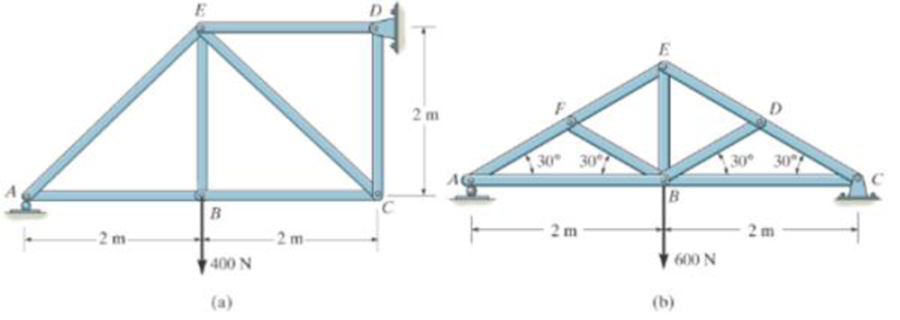#### Videos

Textbook QuestionChapter 6.3, Problem 1PP

In each case, calculate the support reactions and then draw the free-body diagrams of joints A, B, and C of the truss.Prob. P6-108:29
Students have asked these similar questions
The multispan girder has two shear plate connections that act as hinges at C and D. The midspan girder CD is simply supported on the cantilevered ends of the left and right girders. Determine the forces in the hinges and the reactions at supports A, B, E, and F. Also draw the shear and moment diagram.
Draw the Free Body Diagram
The sign has a mass of 150kg with center of mass in G. Draw the free body diagram and determine the components along x,y,z of the reaction in ball joint A as well as the tensile strength in wires BC and BD(Caution : they are two different wires). g=9.81m/s²